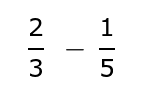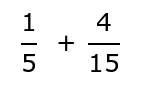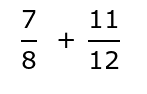# FTCE Number Theory Flashcards

FTCE Number Theory Flashcards
1/55 (missed) 0 0
Create Your Account To Continue Studying

As a member, you'll also get unlimited access to over 79,000 lessons in math, English, science, history, and more. Plus, get practice tests, quizzes, and personalized coaching to help you succeed.

Try it risk-free for 30 days. Cancel anytime
Least Common Multiple
The smallest multiple that a set of numbers share.
Got it
Common Denominator
A number that is a multiple for all of the denominators being considered. Also known as a common multiple.
Got it
Multiple
The product of multiply a number by an integer.
Got it
Composite Number
A number that has more than the two factors of one and itself.
Got it
Determine if 23 is a prime or composite number and explain your choice.
23 is a prime number because it only has the factors one and itself.
Got it
Determine if 21 is a prime or composite number and explain your choice.
21 is a composite number because it has more than two factors. It's factors are 1 x 21 and 3 x 7.
Got it
Determine if 11 is a prime or composite number and explain your choice.
11 is a prime number because it only has the factors one and itself.
Got it
Determine if eight is a prime or composite number and explain your choice.
Eight is a composite number because it has more than two factors. It's factors are 1 x 8, and 2 x 4.
Got it
Explain why one is not a prime number.
A prime number only has the factors one and itself and the number one only has itself as a factors. There are not two distinctly different factors.
Got it
Explain why 0 is not a prime number.
A prime only has the factors one and itself and the number 0 has an infinite amount of primes.
Got it
Fill in the blank with the appropriate number: Other than the number five, no numbers divisible by _____ can be prime numbers.
5
Got it
Determine the only even prime number.
2
Got it
Prime Number
A number that has only two factors: one and itself.
Got it
List the factors of the number -20
1 x -20, -1 x 20, 2 x -10, -2 x 10, 4 x -5, and -4 x 5
Got it
List the factors of the number -8.
-1 x 8, 1 x -8, -2 x 4, and 2 x -4
Got it
List both the positive and negative factors of the number 18.
1 x 18, -1 x -18, 2 x 9, -2 x -9, 3 x 6, and -3 x -6
Got it
List both the positive and negative factors of the number 6.
1 x 6, -1 x -6, 2 x 3, and -2 x -3
Got it
List the positive factors of the number 12.
1 x 12, 2 x 6, and 3 x 4
Got it
List the positive factors of the number 10.
1 x 10 and 2 x 5
Got it
Factors
Two numbers you multiply together to get another number.
Got it

or choose a specific lesson: See all lessons in this chapter
55 cards in set

## Flashcard Content Overview

Number theory involves the study of the relationship between numbers. This necessary understanding of numbers on their most basic level is a vital step towards identifying and analyzing more complex relationships later on in math. First you must be able to identify numbers as even or odd. You then must be able to break a number down from its factor pairs into its most basic prime factors. To do this, it is helpful to understand the rules of divisibility from two up until ten. The last topic this set reviews is the idea of a number's multiples and how that assists a student to find common denominators necessary for fraction operations. This set is to serve as an overview of the number theory concepts and more practice might be necessary based upon your individual needs. Be sure to do a more focused study on those topics when needed.

Front
Back
Factors
Two numbers you multiply together to get another number.
List the positive factors of the number 10.
1 x 10 and 2 x 5
List the positive factors of the number 12.
1 x 12, 2 x 6, and 3 x 4
List both the positive and negative factors of the number 6.
1 x 6, -1 x -6, 2 x 3, and -2 x -3
List both the positive and negative factors of the number 18.
1 x 18, -1 x -18, 2 x 9, -2 x -9, 3 x 6, and -3 x -6
List the factors of the number -8.
-1 x 8, 1 x -8, -2 x 4, and 2 x -4
List the factors of the number -20
1 x -20, -1 x 20, 2 x -10, -2 x 10, 4 x -5, and -4 x 5
Prime Number
A number that has only two factors: one and itself.
Determine the only even prime number.
2
Fill in the blank with the appropriate number: Other than the number five, no numbers divisible by _____ can be prime numbers.
5
Explain why 0 is not a prime number.
A prime only has the factors one and itself and the number 0 has an infinite amount of primes.
Explain why one is not a prime number.
A prime number only has the factors one and itself and the number one only has itself as a factors. There are not two distinctly different factors.
Determine if eight is a prime or composite number and explain your choice.
Eight is a composite number because it has more than two factors. It's factors are 1 x 8, and 2 x 4.
Determine if 11 is a prime or composite number and explain your choice.
11 is a prime number because it only has the factors one and itself.
Determine if 21 is a prime or composite number and explain your choice.
21 is a composite number because it has more than two factors. It's factors are 1 x 21 and 3 x 7.
Determine if 23 is a prime or composite number and explain your choice.
23 is a prime number because it only has the factors one and itself.
Composite Number
A number that has more than the two factors of one and itself.
Multiple
The product of multiply a number by an integer.
Common Denominator
A number that is a multiple for all of the denominators being considered. Also known as a common multiple.
Least Common Multiple
The smallest multiple that a set of numbers share.
Prime Factorization
A number broken down into the product of its prime factors.
List the first four multiples of the number four.
4, 8, 12, and 16
List the first four multiples of the number 11.
11, 22, 33, and 44
Determine the least common multiple for the numbers four and eight.
8
Determine the least common multiple for the numbers four and six.
12
Determine the least common multiple for the numbers five and seven.
35
Write the prime factorization for the number 9.
3 x 3
Write the prime factorization for the number 12.
2 x 2 x 3
Write the prime factorization for the number 30.
2 x 3 x 5
Determine the smallest common denominator:15
Determine the smallest common denominator:15
Determine the smallest common denominator:24
Even Number
Numbers that can be divided by 2 equally.
Odd Number
Numbers that cannot be divided by 2 equally.
Describe how you can determine if a very large number is even or odd without using division.
If the last digit is even, then the entire number is even. If the last digit is odd, then the entire number is odd.
Determine if the number is even or odd: 1,567
Odd
Determine if the number is even or odd: 222,221
Odd
Determine if the number is even or odd: 456,190
Even
Determine if the number is even or odd: 34,986
Even
Fill in the blank with the word even or odd: Zero is NOT an _____ number.
Odd
Divisibility
Being able to be divided equally by a number.
Describe how you know a number is divisible by two.
If you can split the items into two equal groups. You can also tell if a number is divisible by two if the number is even.
Describe how you know a number is divisible by three.
If you can split the items into three groups equally. You can also determine a number is divisible by three if the sum of the digits is divisible by three.
Describe how you know a number is divisible by four.
If you can split the items into four groups equally. You can also determine a larger number is divisible by four, if the number the last two digits create is divisible by four as well.
Describe how you know a number is divisible by five.
If you can split the items into five groups equally. If the number ends in a zero or five, the number is also divisible by five.
Describe how you know a number is divisible by six.
If you can split the items into six groups equally. You can also determine if larger numbers are divisible by six if they are even and follow the rule for divisibility by three as well.
Describe how you know a number is divisible by seven.
If you can split the items into seven groups equally.
Describe how you know a number is divisible by eight.
If you can split the items into eight groups equally. You can determine if larger numbers are divisible by eight if the number created by the last three digits are divisible by eight.
Describe how you know a number is divisible by nine.
If you can split the items into nine groups equally. You can also determine if a larger number is divisible by nine if the sum of the digits is divisible by nine as well.
Describe how you know a number is divisible by 10.
If you can split the items into ten groups equally. If the last digit is zero, the number is also divisible by 10.
Determine if the number 12 is divisible by 2, 3, 4, 5, 6, 7, 8, 9, and/or 10.
The number 12 is only divisible by 2, 3, and 6.
Determine if the number 30 is divisible by 2, 3, 4, 5, 6, 7, 8, 9, and/or 10.
The number 30 is only divisible by the numbers 2, 3, 5, and 10.
Determine if the number 32 is divisible by 2, 3, 4, 5, 6, 7, 8, 9, and/or 10.
The number 32 is only divisible by the numbers 2, 4, and 8.
Determine if the number 35 is divisible by 2, 3, 4, 5, 6, 7, 8, 9, and/or 10.
The number 35 is only divisible by the numbers 5 and 7.
Determine if the number 27 is divisible by 2, 3, 4, 5, 6, 7, 8, 9, and/or 10.
The number 27 is only divisible by the numbers 3 and 9.

To unlock this flashcard set you must be a Study.com Member.